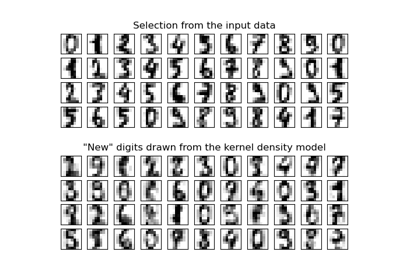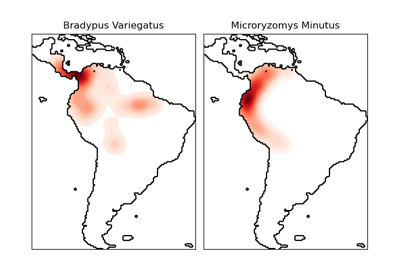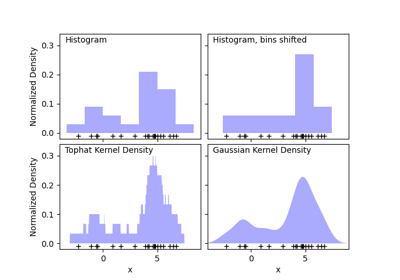# sklearn.neighbors.KernelDensity¶

class sklearn.neighbors.KernelDensity(*, bandwidth=1.0, algorithm='auto', kernel='gaussian', metric='euclidean', atol=0, rtol=0, breadth_first=True, leaf_size=40, metric_params=None)[source]

Kernel Density Estimation.

Read more in the User Guide.

Parameters
bandwidthfloat

The bandwidth of the kernel.

algorithmstr

The tree algorithm to use. Valid options are [‘kd_tree’|’ball_tree’|’auto’]. Default is ‘auto’.

kernelstr

The kernel to use. Valid kernels are [‘gaussian’|’tophat’|’epanechnikov’|’exponential’|’linear’|’cosine’] Default is ‘gaussian’.

metricstr

The distance metric to use. Note that not all metrics are valid with all algorithms. Refer to the documentation of BallTree and KDTree for a description of available algorithms. Note that the normalization of the density output is correct only for the Euclidean distance metric. Default is ‘euclidean’.

atolfloat

The desired absolute tolerance of the result. A larger tolerance will generally lead to faster execution. Default is 0.

rtolfloat

The desired relative tolerance of the result. A larger tolerance will generally lead to faster execution. Default is 1E-8.

If true (default), use a breadth-first approach to the problem. Otherwise use a depth-first approach.

leaf_sizeint

Specify the leaf size of the underlying tree. See BallTree or KDTree for details. Default is 40.

metric_paramsdict

Additional parameters to be passed to the tree for use with the metric. For more information, see the documentation of BallTree or KDTree.

sklearn.neighbors.KDTree

K-dimensional tree for fast generalized N-point problems.

sklearn.neighbors.BallTree

Ball tree for fast generalized N-point problems.

Examples

Compute a gaussian kernel density estimate with a fixed bandwidth. >>> import numpy as np >>> rng = np.random.RandomState(42) >>> X = rng.random_sample((100, 3)) >>> kde = KernelDensity(kernel=’gaussian’, bandwidth=0.5).fit(X) >>> log_density = kde.score_samples(X[:3]) >>> log_density array([-1.52955942, -1.51462041, -1.60244657])

Methods

 fit(X[, y, sample_weight]) Fit the Kernel Density model on the data. get_params([deep]) Get parameters for this estimator. sample([n_samples, random_state]) Generate random samples from the model. score(X[, y]) Compute the total log probability density under the model. Evaluate the log density model on the data. set_params(**params) Set the parameters of this estimator.
__init__(*, bandwidth=1.0, algorithm='auto', kernel='gaussian', metric='euclidean', atol=0, rtol=0, breadth_first=True, leaf_size=40, metric_params=None)[source]

Initialize self. See help(type(self)) for accurate signature.

fit(X, y=None, sample_weight=None)[source]

Fit the Kernel Density model on the data.

Parameters
Xarray_like, shape (n_samples, n_features)

List of n_features-dimensional data points. Each row corresponds to a single data point.

yNone

Ignored. This parameter exists only for compatibility with sklearn.pipeline.Pipeline.

sample_weightarray_like, shape (n_samples,), optional

List of sample weights attached to the data X.

New in version 0.20.

Returns
selfobject

Returns instance of object.

get_params(deep=True)[source]

Get parameters for this estimator.

Parameters
deepbool, default=True

If True, will return the parameters for this estimator and contained subobjects that are estimators.

Returns
paramsmapping of string to any

Parameter names mapped to their values.

sample(n_samples=1, random_state=None)[source]

Generate random samples from the model.

Currently, this is implemented only for gaussian and tophat kernels.

Parameters
n_samplesint, optional

Number of samples to generate. Defaults to 1.

random_stateint, RandomState instance, default=None

Determines random number generation used to generate random samples. Pass an int for reproducible results across multiple function calls. See :term: Glossary <random_state>.

Returns
Xarray_like, shape (n_samples, n_features)

List of samples.

score(X, y=None)[source]

Compute the total log probability density under the model.

Parameters
Xarray_like, shape (n_samples, n_features)

List of n_features-dimensional data points. Each row corresponds to a single data point.

yNone

Ignored. This parameter exists only for compatibility with sklearn.pipeline.Pipeline.

Returns
logprobfloat

Total log-likelihood of the data in X. This is normalized to be a probability density, so the value will be low for high-dimensional data.

score_samples(X)[source]

Evaluate the log density model on the data.

Parameters
Xarray_like, shape (n_samples, n_features)

An array of points to query. Last dimension should match dimension of training data (n_features).

Returns
densityndarray, shape (n_samples,)

The array of log(density) evaluations. These are normalized to be probability densities, so values will be low for high-dimensional data.

set_params(**params)[source]

Set the parameters of this estimator.

The method works on simple estimators as well as on nested objects (such as pipelines). The latter have parameters of the form <component>__<parameter> so that it’s possible to update each component of a nested object.

Parameters
**paramsdict

Estimator parameters.

Returns
selfobject

Estimator instance.

## Examples using sklearn.neighbors.KernelDensity¶